### Choose language# Code and calculation settings in RCS

$$Project dataFirst, let's start with the Project data settings. You can access the table from the top ribbon -> Settings toolbar. One of the things to be defined here is the base information about the project like project name, number, description, author, and date. This information will be then sent to the automatic report. Another input you can do here is the settings of the default values for the National Code. You can set the codes and national annexe for the project as well as functionalities, design working life, exposure classes, and material grades.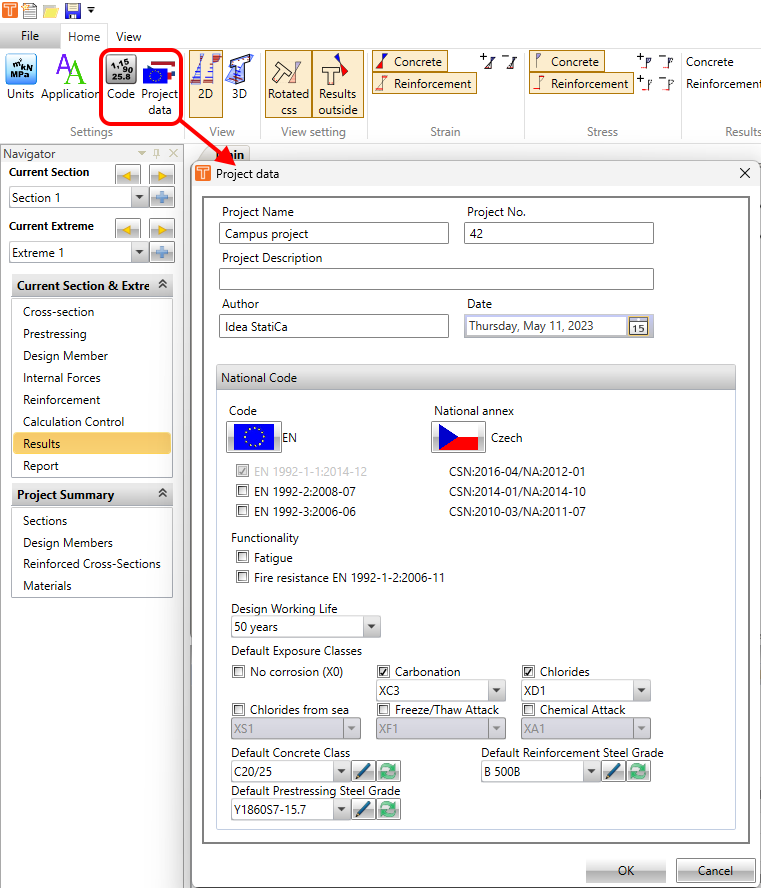Code and calculation settingsSecondly, there are the Code and calculation settings where you can go through the chapters of the used code and change the default values of coefficients, limits, or conditions.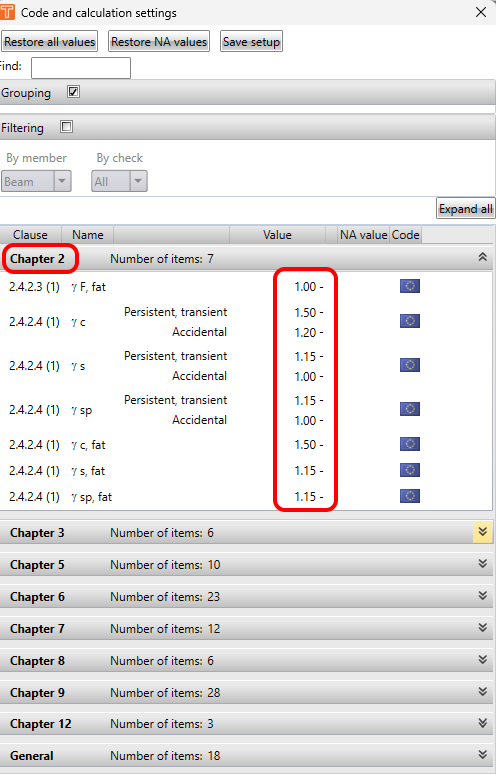If the national annexe is selected, you will see the flag of the annexe next to the values which differ from the general Eurocode. There will be also the new value or for example the equation for the automatic calculation. Like it is shown in the following figure.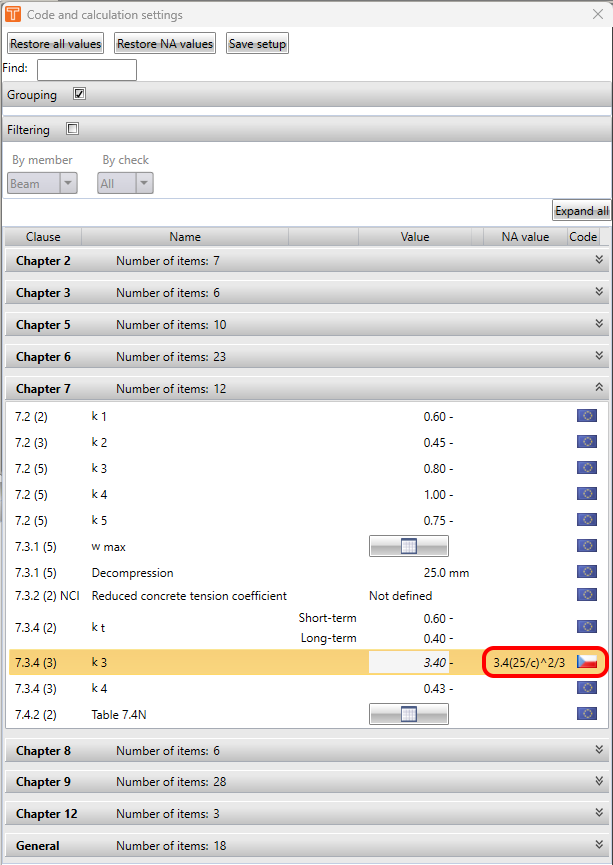There can be also a table input that can be of course adjusted in the same way. See the example with the table of the crack width in the next figure.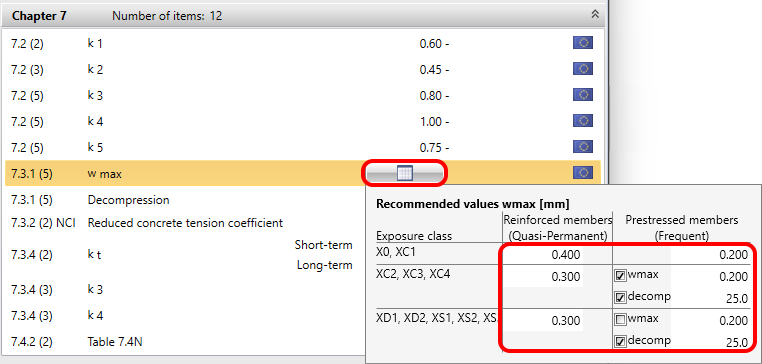The last but not least tab is the General. Here are the general settings of the calculation.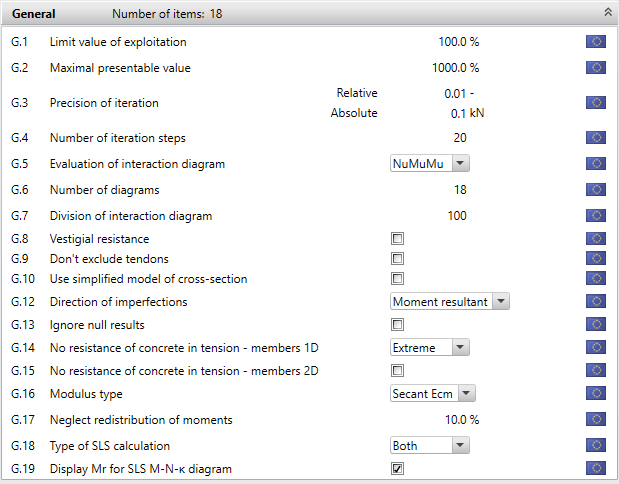Let's go through them: G.1 - Limit value of exploitation - The maximal value of the utilization of the cross-section doesn't have to be 100%. You can change it by using this settingG.2 - Maximal presentable value - Here you can change the maximal value of the utilization.G.3 and G.4 - Precision of iteration and Number of iteration steps - You can also influence the iteration process. It can help with the speed of the calculation.G.5 - Evaluation of iteration diagram - It is described in the following article in the chapter Capacity N-M-M: ULS results in RCSG.6 - Number of diagrams - Number of vertical sections through interaction surface around vertical axes. If you increase the number you can increase the precision of the interaction diagram. Read more in the following article: BendingG.7 - Division of interaction diagram - Again if you increase the number you can increase the precision of the interaction diagram.G.8 - Vestigial resistance - It is valid for prestressed cross-sections only. If it is turned OFF - The left-hand side of the reliability condition contains the external load only. The right-hand side is the resistance, which consists of the primary effects resulting from the decompression prestressing force and the effect of the vestigial resistance of the prestressing reinforcement. If it is turned ON - An additional table is displayed, in which the left-hand side of the reliability condition contains the external load minus the primary effect resulting from the decompression prestressing force. The right-hand side is the resistance, which consists of the effect of the vestigial resistance of the prestressing reinforcement only.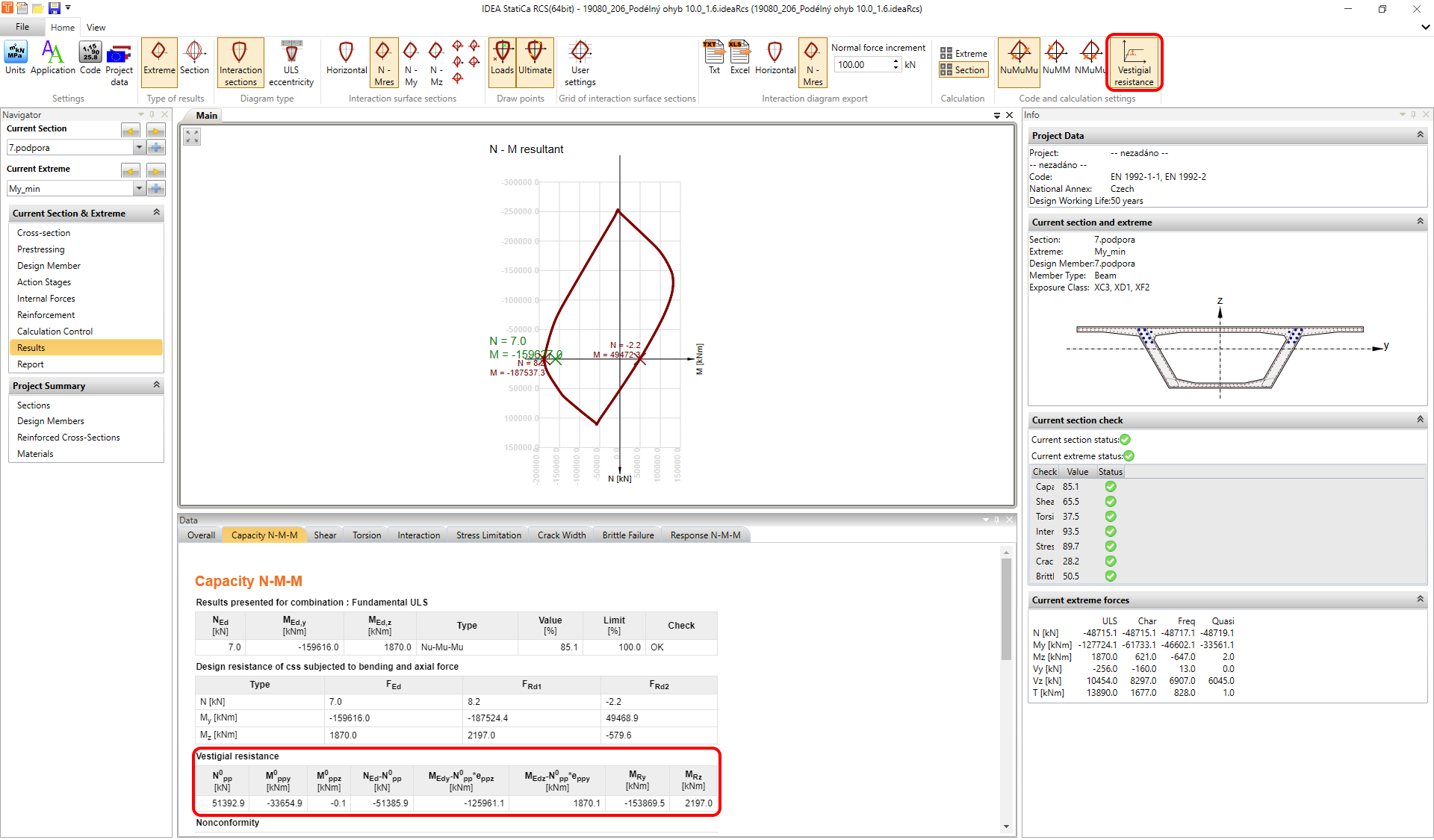G.9 - Don't exclude tendons - If it is turned on the tendons which are outside of the cross-section will be included in the calculation.G.10 - Used a simplified model - You can use this option to speed up your calculation.G.12 - Direction of imperfection - The effect is described in the following article: Second-order effect in RCS applicationG.14 - No resistance of concrete in tension - members 1D Always - The assumption that the concrete resists no tension will be applied in all SLS checks for all combinations of all sections and their extremes. Section - In the case that the upper or lower design value of internal forces of one of the SLS combinations will cause concrete stress higher than the concrete tensile strength, the assumption that the concrete resists no tension will be applied in all SLS checks for all extremes of the current section. The assumptions for SLS checks in combinations of other sections will not be influenced. Extreme - In the case that the upper or lower design value of internal forces of one of the SLS combinations will cause concrete stress higher than the concrete tensile strength, the assumption that the concrete resists no tension will be applied in all SLS checks for current extreme only. The assumptions for SLS checks in other extremes of the current section will not be influenced. G.15 - No resistance of concrete in tension - members 2D - ON tensile in concrete for SLS checks is neglected. OFF the calculation is provided according to the code.G.16 - Modulus type - You can select the type of the modulus of elasticity used for long-term SLS checks Secant Ecm Tangent Ec = 1,05*Ecm G.17 - Neglect redistribution of momentsG.18 - Type of SLS calculation - By this setting, you can calculate only long-term or short-term checks for SLSG19 - Display Mr for SLS M-N-κ diagram$$